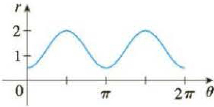Chapter 10.3, Problem 47E

Chapter
Section
Textbook Problem

# The figure shows a graph of r as a function of θ in Cartesian coordinates. Use it to sketch the corresponding polar curve.47.To determine

To sketch: Polar curve from the given Cartesian curve shown below in figure 1.

Explanation

Given:

The polar curve is shown below in figure 1.

Calculation:

From the figure 1, when θ increases from 0 to π2 , polar curve r increases from 0.5 to 2 in polar graph.

When θ increases from π2 to π , polar curve r decreases from 2 to 0.5

### Still sussing out bartleby?

Check out a sample textbook solution.

See a sample solution

#### The Solution to Your Study Problems

Bartleby provides explanations to thousands of textbook problems written by our experts, many with advanced degrees!

Get Started

#### Find more solutions based on key concepts